Learn all Concepts of Chapter 3 Class 10 (with VIDEOS). Check - Linear Equations in 2 Variables - Class 10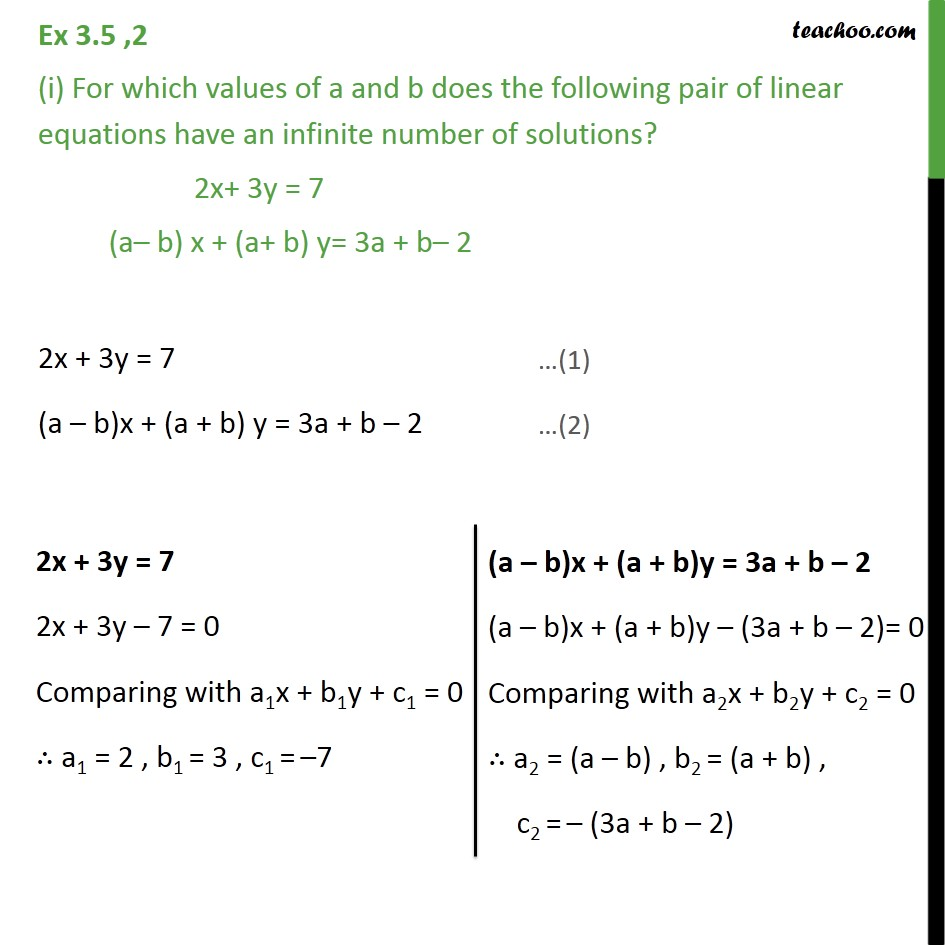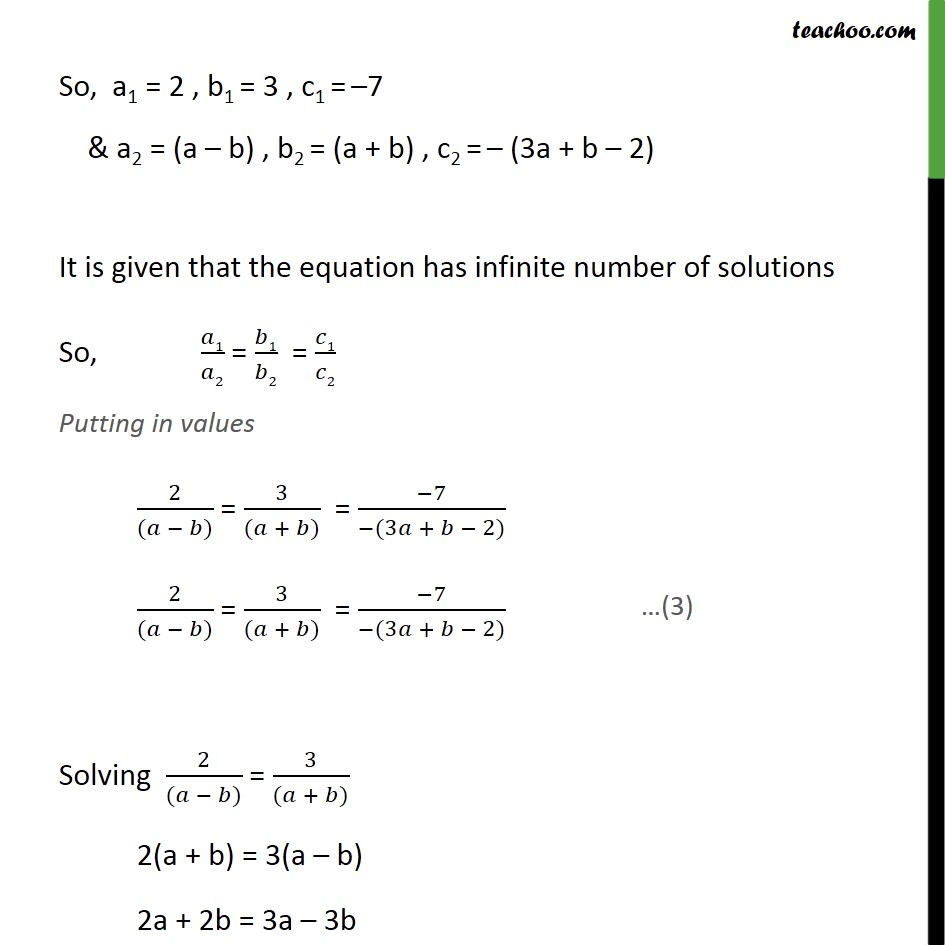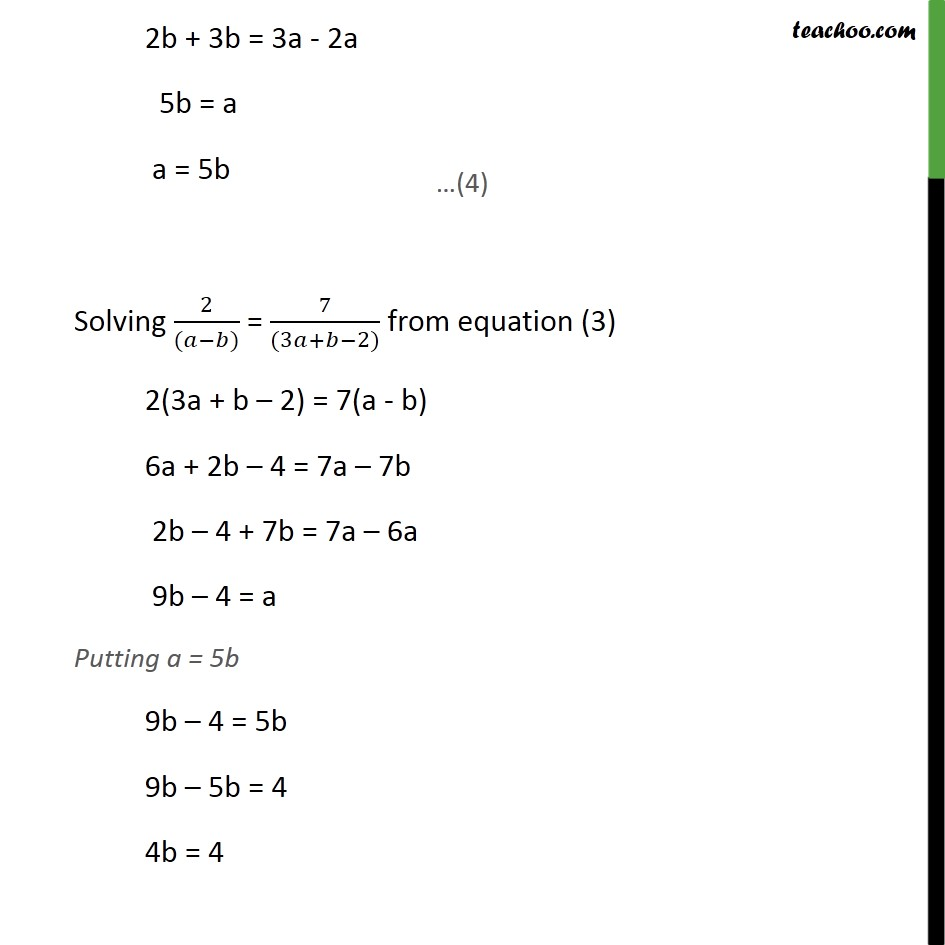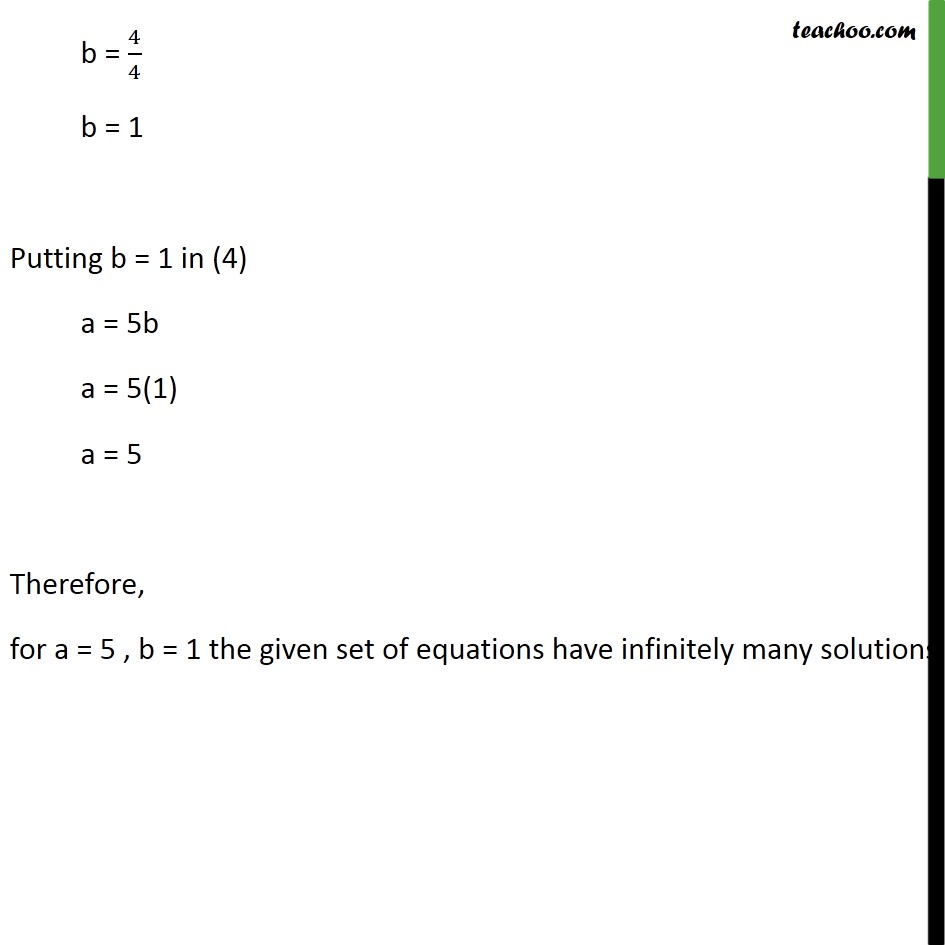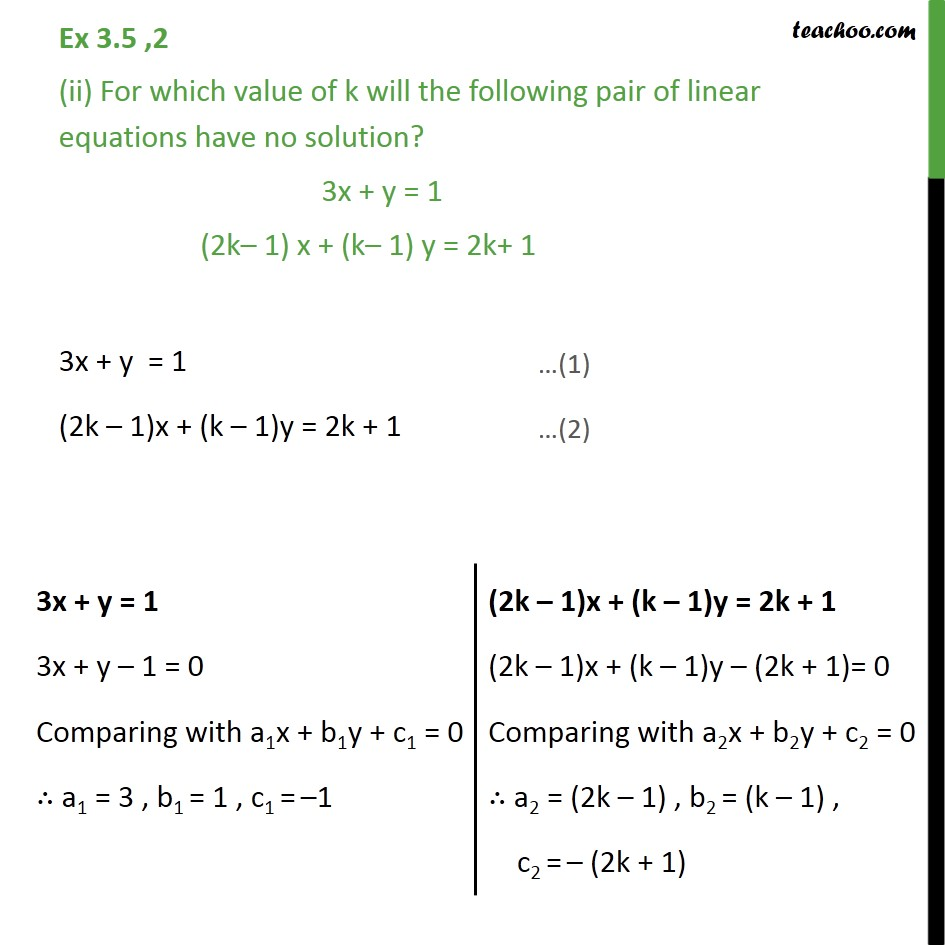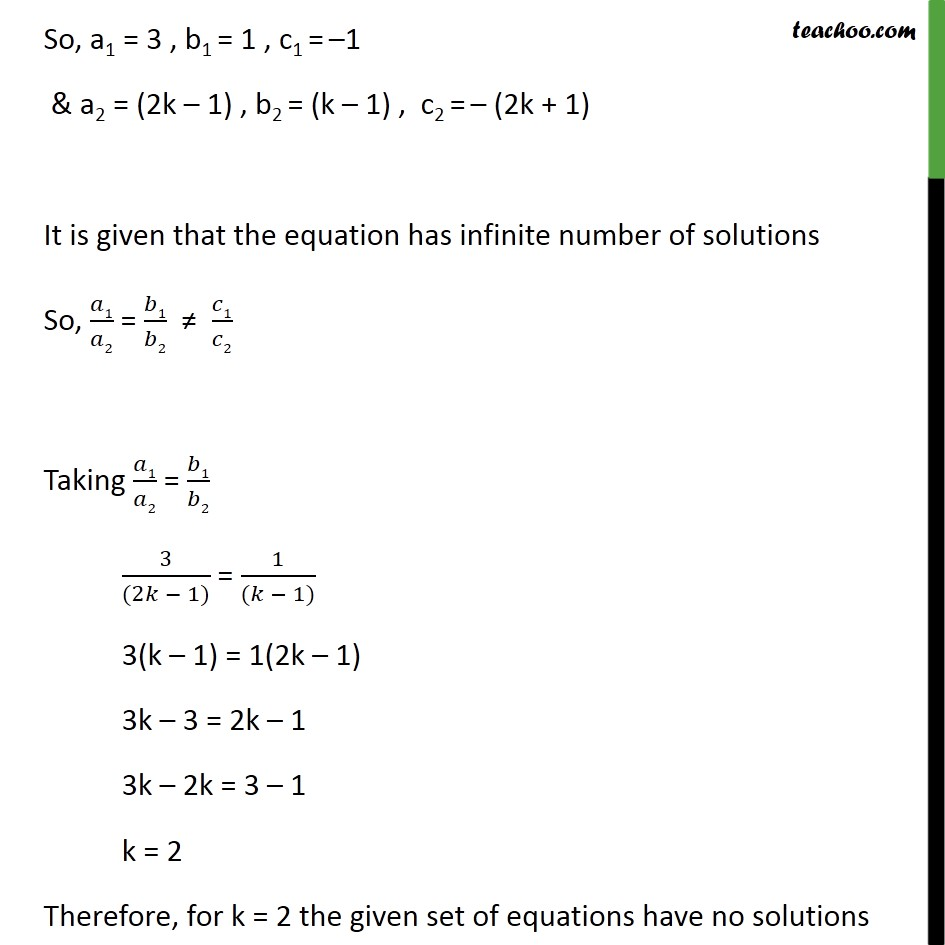1. Chapter 3 Class 10 Pair of Linear Equations in Two Variables
2. Serial order wise
3. Ex 3.5

Transcript

Ex 3.5 ,2 (i) For which values of a and b does the following pair of linear equations have an infinite number of solutions? 2x+ 3y = 7 (a b) x + (a+ b) y= 3a + b 2 2x + 3y = 7 (a b)x + (a + b) y = 3a + b 2 So, a1 = 2 , b1 = 3 , c1 = 7 & a2 = (a b) , b2 = (a + b) , c2 = (3a + b 2) It is given that the equation has infinite number of solutions So, 1/ 2 = 1/ 2 = 1/ 2 Putting in values 2/(( )) = 3/(( + )) = ( 7)/( (3 + 2)) 2/(( )) = 3/(( + )) = ( 7)/( (3 + 2)) Solving 2/(( )) = 3/(( + )) 2(a + b) = 3(a b) 2a + 2b = 3a 3b 2b + 3b = 3a - 2a 5b = a a = 5b Solving 2/(( )) = 7/((3 + 2)) from equation (3) 2(3a + b 2) = 7(a - b) 6a + 2b 4 = 7a 7b 2b 4 + 7b = 7a 6a 9b 4 = a Putting a = 5b 9b 4 = 5b 9b 5b = 4 4b = 4 b = 4/4 b = 1 Putting b = 1 in (4) a = 5b a = 5(1) a = 5 Therefore, for a = 5 , b = 1 the given set of equations have infinitely many solutions Ex 3.5 ,2 (ii) For which value of k will the following pair of linear equations have no solution? 3x + y = 1 (2k 1) x + (k 1) y = 2k+ 1 3x + y = 1 (2k 1)x + (k 1)y = 2k + 1 So, a1 = 3 , b1 = 1 , c1 = 1 & a2 = (2k 1) , b2 = (k 1) , c2 = (2k + 1) It is given that the equation has infinite number of solutions So, 1/ 2 = 1/ 2 1/ 2 Taking 1/ 2 = 1/ 2 3/((2 1)) = 1/(( 1)) 3(k 1) = 1(2k 1) 3k 3 = 2k 1 3k 2k = 3 1 k = 2 Therefore, for k = 2 the given set of equations have no solutions

Ex 3.5